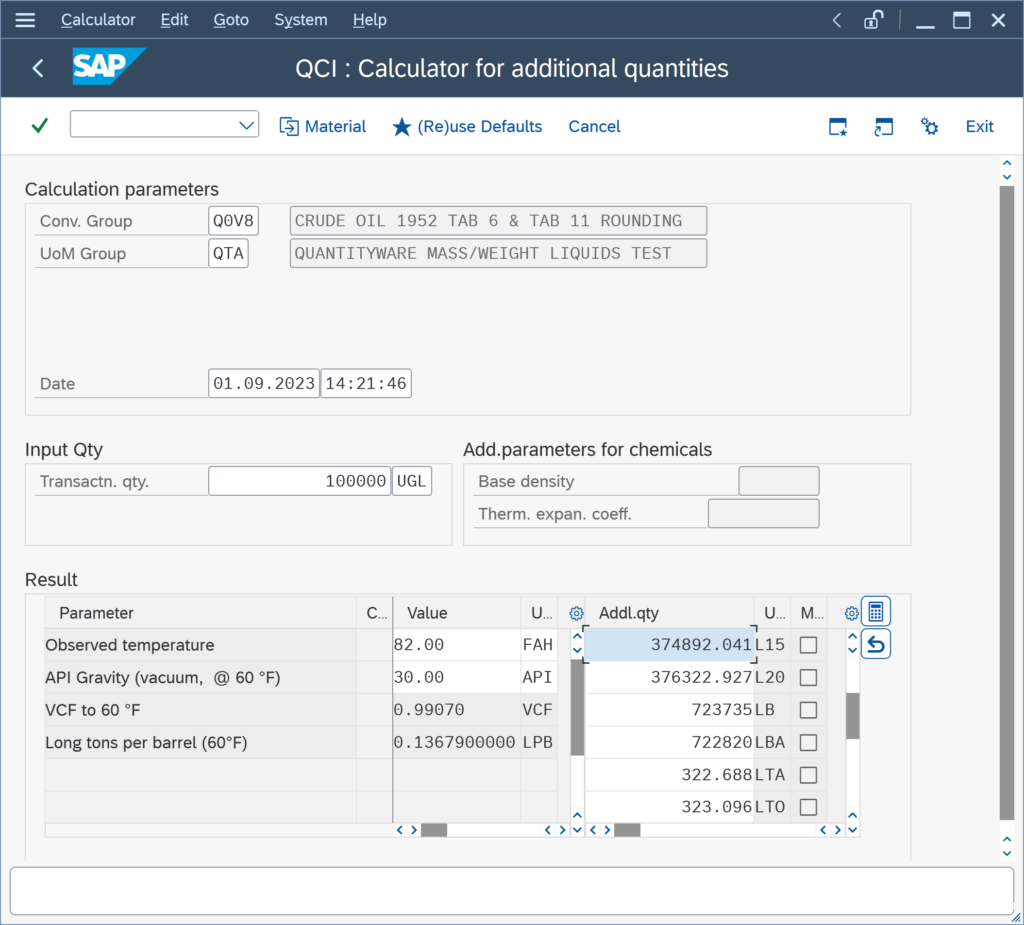Search QuantityWare

# Oil & Gas Quantity Conversion Basics FAQs

Answers to common questions regarding information on basic oil & gas quantity conversion engineering principles

### How are the additional quantity values calculated for a specific conversion group?

You perform a test calculation utilizing transaction O3QCITEST, and you want to manually confirm (e.g. via an XLS) the calculation results:For each conversion group, exactly one calculation model is defined/configured. Details for all models can be found in the BCP Documentation Reference Manual, Chapter 6.4 .
The calculation of the L15, L20, LB, … quantity values is performed sequentially, i.e. for each UoM a defined model calculation sequence is executed. The fundamental concept is, that each conversion group is defined to perform the calculations via a set of so-called Base Conversion Units of Measure.

### How do I use LPG vapor space calculations to determine the amount of LPG transferred?

When transferring LPG, we need to accurately measure the amount transferred. To do this, we make use of LPG vapor space calculations.

The video below describes the details of LPG storage, along with details of how to perform static LPG vapor space calculations using both transferred mass calculation and movement analysis methods, via QuantityWare’s Bulk Calculations Solution (BCS):

Vimeo Video

### How is the density type defined, that we set in a conversion group?

Within the oil industry, three different “density types” for liquid bulk products are commonly in use:

Density values can be expressed in any of these three density types and are related via well-defined formulas. SAP QCI conversion groups require data entry of a density value in the density type as defined in the conversion group; MQCI conversion groups can be configured to allow data entry of density values of any of the three density types.

### How is the API gravity of a liquid bulk product defined?

The API gravity is unique to the oil industry. It is defined via the following equation:

API gravity = [ 141.5 / RD ] – 131.5

RD = relative density of liquid product – see separate FAQ.

Example:

What is the API gravity of the truck load of gasoline with an absolute density of 800 kg/m³ ?

Solution:

• ASTM D1250-80: API = 141.5 / 0.800 791 – 131.5 = 45.20029
• ASTM D1250-04: API = 141.5 / 0.800 788 – 131.5 = 45.20095

(Both values above are rounded to five decimals)

### How is the relative density of a liquid bulk product defined?

The relative density RD of a liquid (formerly also known as “specific gravity”) is defined via the following equation:

RD = ρ / D60

ρ = absolute density of the liquid

D60 = absolute density of water at 60 °F.

The D60 value needs to be known to convert a relative density value into an absolute density value and vice versa.

The D60 value is determined experimentally, and has two values provided via ASTM standards (one valid until 1980, later redefined in 2004):

• ASTM D1250-80: D60 = 999.012 kg/m³
• ASTM D1250-04: D60 = 999.016 kg/m³

The latter is due to changes in the international temperature scale.

### How is the absolute density of a bulk product defined?

The absolute density ρ of a liquid is defined via the following equation:

ρ = m / V

m = mass of the liquid

V = volume which the liquid occupies.

Example:

What is the absolute density ρ of a truck load of gasoline, which has a mass of 1,200 kg and occupies a volume of 1.5 m³ ?

Solution:

ρ = 1,200 kg / 1.5 m³ = 800 kg/m³

Note:

Since the volume V which the liquid occupies changes with the liquid temperature,

### How can I change the number of decimals (rounding) of my VCF / CTL ?

The Volume Correction Factor (VCF), also known as Correction for the effect of Temperature on Liquid (CTL), is defined in various measurement standards – whereby product specific standards and historical versions need to be considered. Therein, the calculation procedure and the number of decimals, to which the VCF shall be rounded, are specified.

Historically, volume correction factors have been defined as printed tables – starting with ASTM D1250-80, implementation procedures for software programs became the primary standard, not the printed tables any more.

Examples: Printed tables print the VCF with 3 or 4 decimals (e.g.